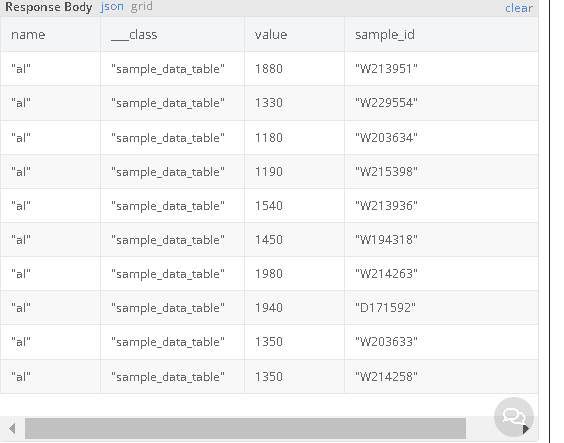# How to write nested query

I have a table (sample_data) with columns sample_id, element, value

I’m using a load table objects block and want to return sample_id such that several element:value pairs are true.
for example return sample_id if al<3500 and ca<600

I tried this

sample_id in ( name=‘al’ AND value<‘3500’) and sample_id in ( name=‘ca’ AND value<‘600’)

but got this error back

Where clause ’ sample_id IN ( name=‘al’ AND value<‘3500’) AND sample_id IN ( name=‘ca’ AND value<‘600’)’ is invalid

how should I be doing this?

as always thanks for your time.
H

Hi @hharrington,

Did I understand correctly that you need to return the sample_id property for those objects that match this where clause `name='al' AND value<'3500'`?
What is `name`? Is it another column? Please provide us with the logic you already have.

Regards,
Marina

oh so sorry ( doing two things at once )

this is the logic I have but it errors out and returns nothing

``````sample_id in ( element=‘al’ AND value<‘3500’) and sample_id in ( name=‘ca’ AND value<‘600’)
``````

Where clause ’ sample_id IN ( element=‘al’ AND value<‘3500’) AND sample_id IN ( element=‘ca’ AND value<‘600’)’ is invalid

yes I need to return the sample_ids if both element=‘al’ AND value<‘3500’ AND sample_id IN ( element=‘ca’ AND value<‘600’)

IE both statements are true. I am also add more terms.

very sorry for my typo

H

The syntax for subquery you’re using is not supported. Please see the documentation for the format Backendless recognizes:
https://backendless.com/docs/rest/data_search_with_subquery.html#general-subquery-syntax

Here’s a video on sub queries, you might find it helpful:

Regards,
Mark

Thanks for the response. that video talks about a query across two tables, what I’m wanting to do is make a query on a single table.

I really did a bad job of describing what I’m trying to do so let me give more detail.

I have a table sample_data it has 3 columns sample_id, element, value ( well is has a forth column which is computed as a cancat of sample_id and element and is set to unique so there will only be one entry of an element for a sample )

sample_id is a soil sample and element a trace element found in the sample with its value (parts-per-million)

say we are tracking 10 elements, each sample_id will be in the table 10 times one entry for each element being tracked.

thus rows will look like this:
xxxxx, al, 2300
if I am tracking 10 elements there will be 10 rows for xxxxx each with a different element

so I need to return a list of sample_id where element ( say Al for aluminum ) < 2000 and element (say Ca calcium ) < 600

I want to return every sample_id where both those things are true.

I hope this make more sense.

H

The following query would do it:

``````(name = 'al' and value < 2000) or (name = 'ca' and value < 600)
``````
1 Like

ok if I set the properties in the load table block to “sameple_id”

it looks like (name = ‘al’ and value < 2000) or (name = ‘ca’ and value < 600)

will return all sample_ids where al is < 2000 and also where ca is < 600
so a sample_id where al is < 2000 but the ca value was 2000 it would also be returned.

I need to return only sample_id’s where both those are true

looking up how to do this for mysql I found this

``````SELECT DISTINCT asset_id FROM asset_keyword_table WHERE
( category_id = 4424 AND keyword_id = 2 AND value LIKE '%abc%' )
AND asset_id IN
( SELECT DISTINCT asset_id FROM asset_keyword_table WHERE
category_id = 4424 AND keyword_id = 3 AND value LIKE '%Jpeg%' )
``````

but the load table block does not seem to have a way to reference the thing you are selecting (since its put in the properties field of that block.

H

Could you run the query in REST console, select the Response to render as a grid and show me which records do not match the query?

sure

here is the rest console set up

here is the responselooking at the first item returned, W213951

and using this “sample_id=‘W213951’ and (name=‘al’ or name=‘ca’)” as the search criteria I see

showing that W213951 entry for name ‘ca’ is 801 which is higher than 600, so I’d want W213951 excluded from the results.

Note: in my messing about I changed column ‘element’ (referenced in my first post ) to ‘name’

thanks again for taking a look at this.

H

Hello @hharrington,

We’re investigating this question.

Hi @hharrington ,

It seems that I found a solution which may work for your case.

Here is an example of such query:

In this query I searched `sample_id` by condition which includes presense of 2 entries. Pay attention to the `having` clause - it filters out all `sample_id` which have less than 2 entries.

In your case, number in `having` clause should always match number of elements which should be present in your sample.

If you have any further questions post them here and I will try to help.

Regards, Andriy

EDIT: removed last response as I just was your entry
I will test now

Ok that seems promising how do a set that up in with the load table objects?

I can see there is a having clause and group by but don’t see where I would place the count function

is there a link you can give me or can you point me to an image of how this should be done?

thanks,
H

Count would be just a property added like this:

``````count(name) as amount_of_entries_for_sample
``````

Thanks I’ll test this out!

H